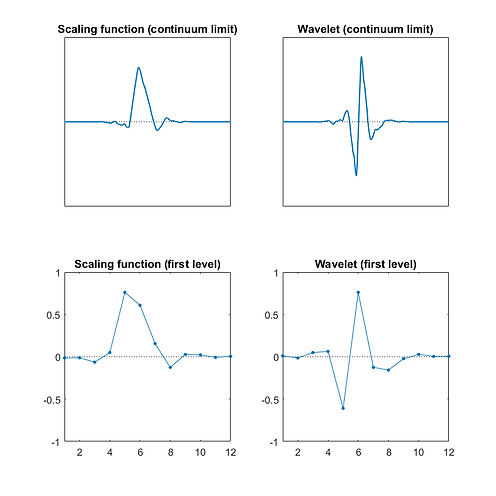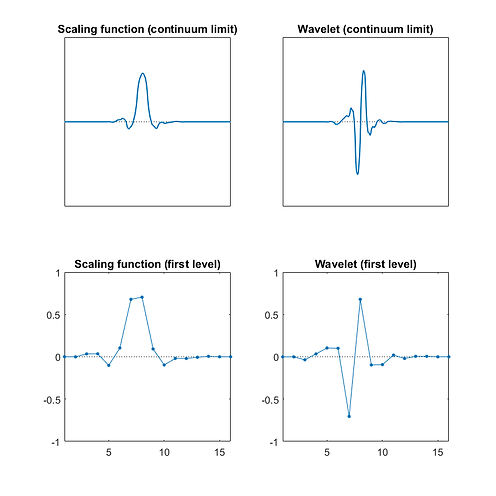EN
FR

## Multiwavelets 4-4

• orthogonal

• dilation factor 2

• two wavelets Wl and Wr at each scale (related through spatial reflection)

• two scaling functions Sl and Sr at each scale (related through spatial reflection)

• built from products of (reflection symmetric) 4×4 unitary matrices

• optimized to maximize the vanishing moments of the wavelets

• similar to CL multiwavelets (but not quite the same)

General notes:​ Depicted right is the unitary circuit representation of the depth 3 circuit (i.e with 3 sub-layers of unitary gates in each layer). The top sub-layer in each layer is formed from unitary gates u, while all subsequent layers are formed from unitary gates v. Tables of the angles θij parametrizing these gates are presented below. Notice that these angles initially change with scale i, but converge to fixed values for larger scales (i.e. higher layers in the circuit).

Also presented below are the l.h.s facing scaling functions Sl and wavelets Wl from different depth circuits within this family. Alternatively one could form symmetric Ws and anti-symmetric Wa wavelets through linear combinations: Ws = (Wl + Wr) and Wa = (Wl - Wr). Notice, however, that in many instances the wavelets Wr and Wl are already very close to being anti-symmetric.MATLAB code for constructing these wavelets and demonstrating their use in image compression can be found here:

Depth 1 circuit (2 vanishing moments)

Circuit angles θijDepth 2 circuit (3 vanishing moments)

Circuit angles θijDepth 3 circuit (4 vanishing moments)

Circuit angles θijDepth 4 circuit (5 vanishing moments)

Circuit angles θijCircuit angles θij

Depth 5 circuit (6 vanishing moments)